# 908. Smallest Range I 最小差值 I

@TOC

## # 题目描述

Given an array A of integers, for each integer A[i] we may choose any x with -K <= x <= K, and add x to A[i].

After this process, we have some array B.

Return the smallest possible difference between the maximum value of B and the minimum value of B.

Example 1:

Input: A = , K = 0
Output: 0
Explanation: B = 


Example 2:

Input: A = [0,10], K = 2
Output: 6
Explanation: B = [2,8]


Example 3:

Input: A = [1,3,6], K = 3
Output: 0
Explanation: B = [3,3,3] or B = [4,4,4]


Note:

1. 1 <= A.length <= 10000
2. 0 <= A[i] <= 10000
3. 0 <= K <= 10000

## # 题目大意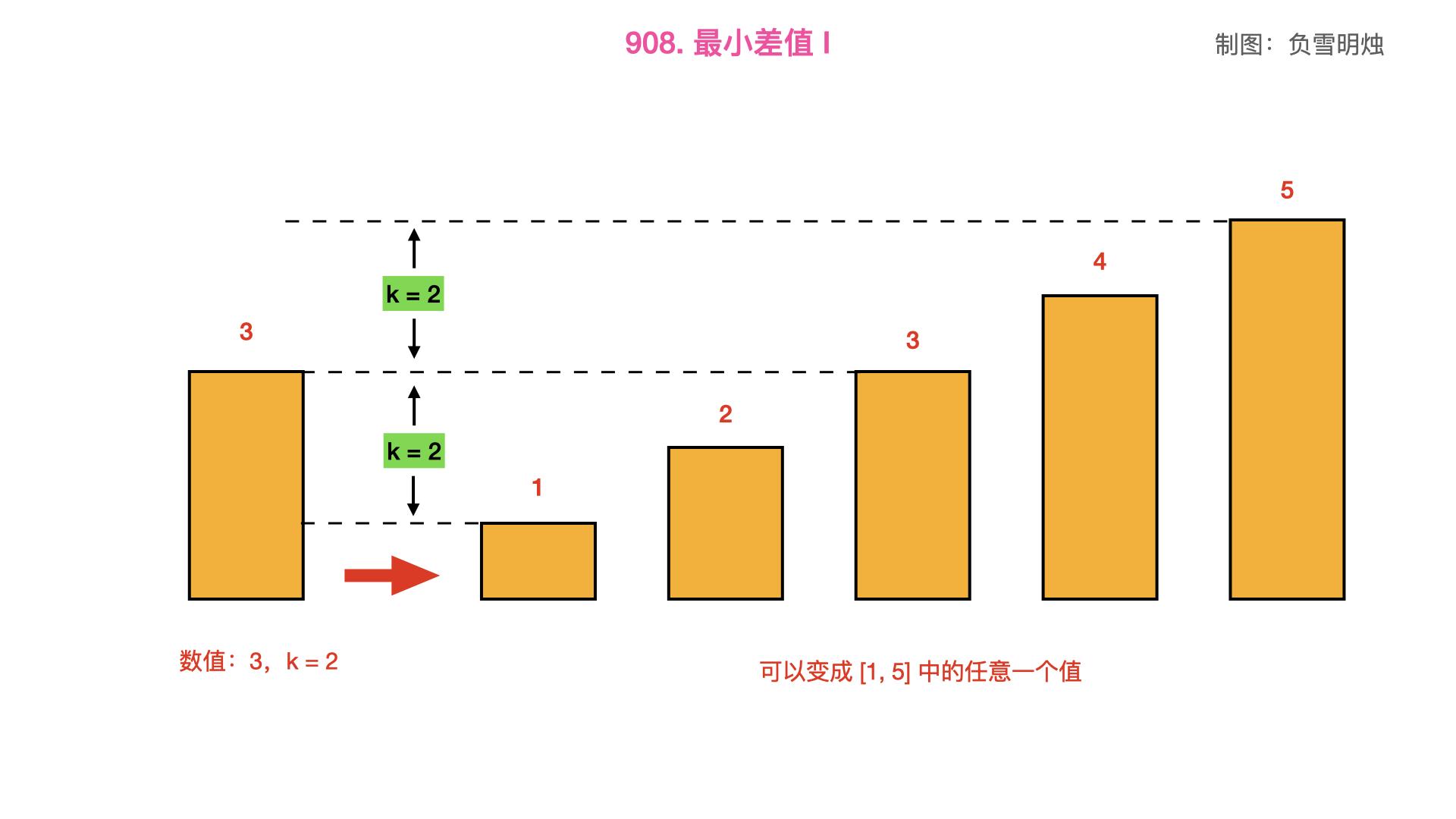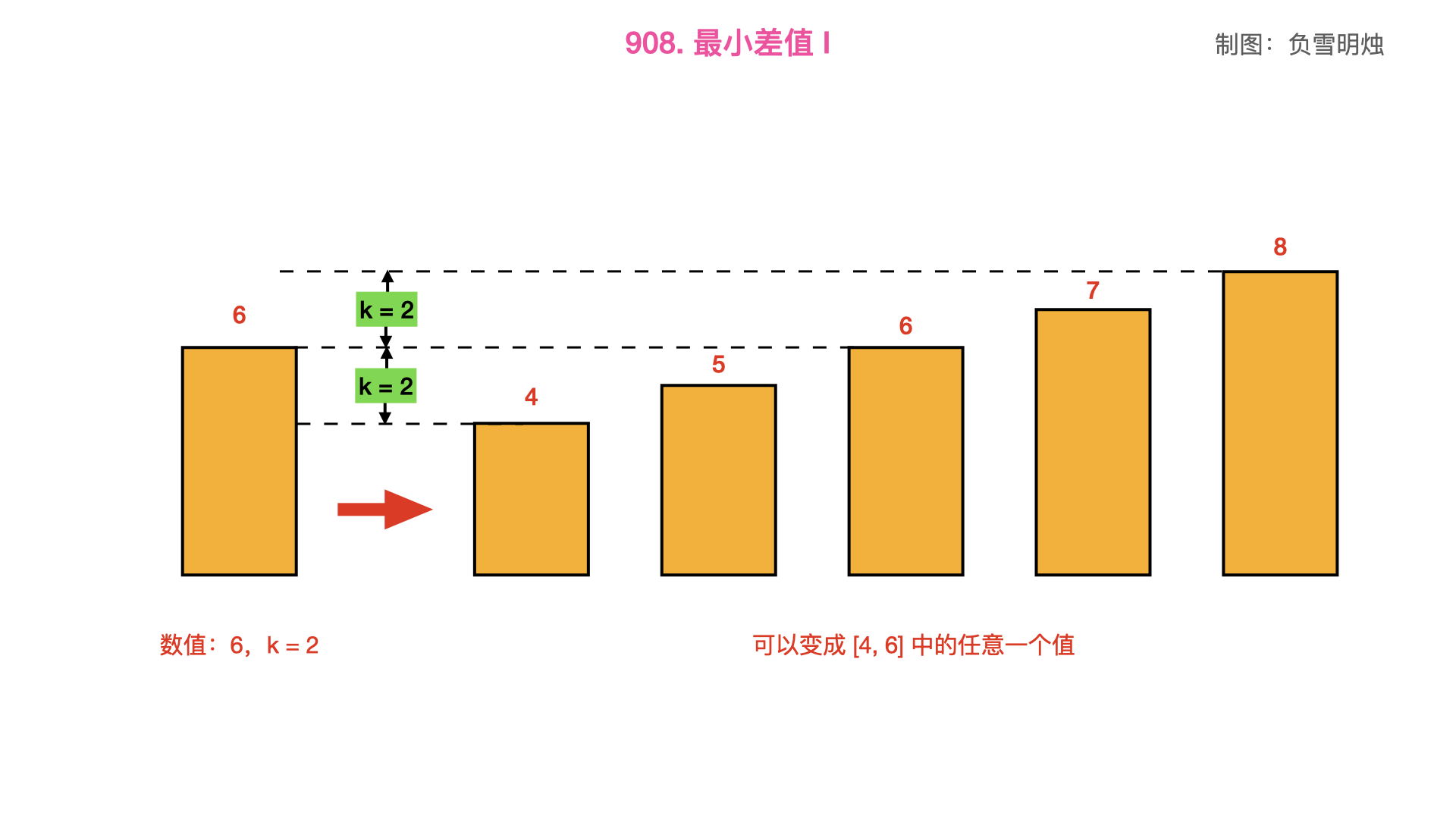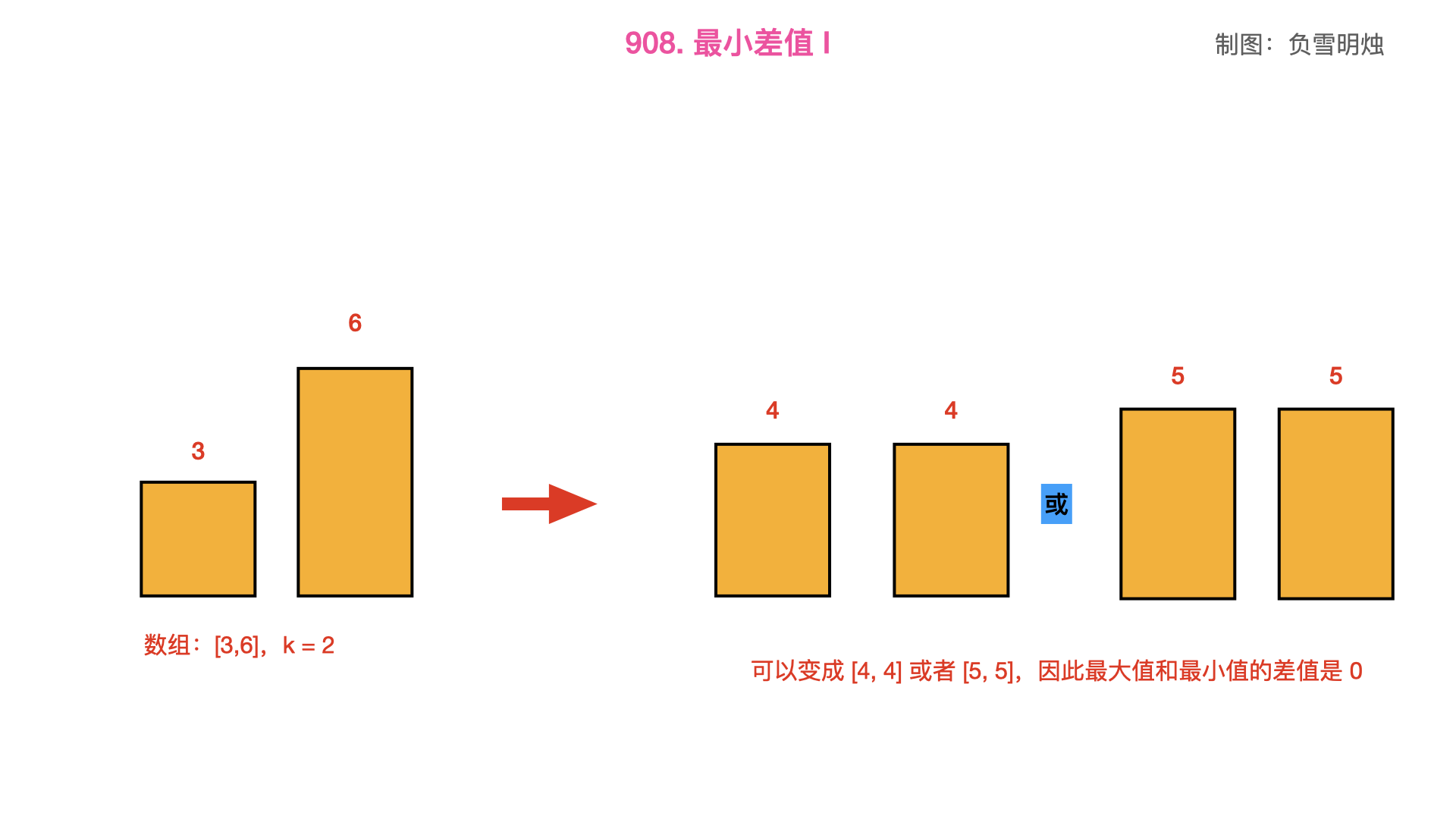## # 解题方法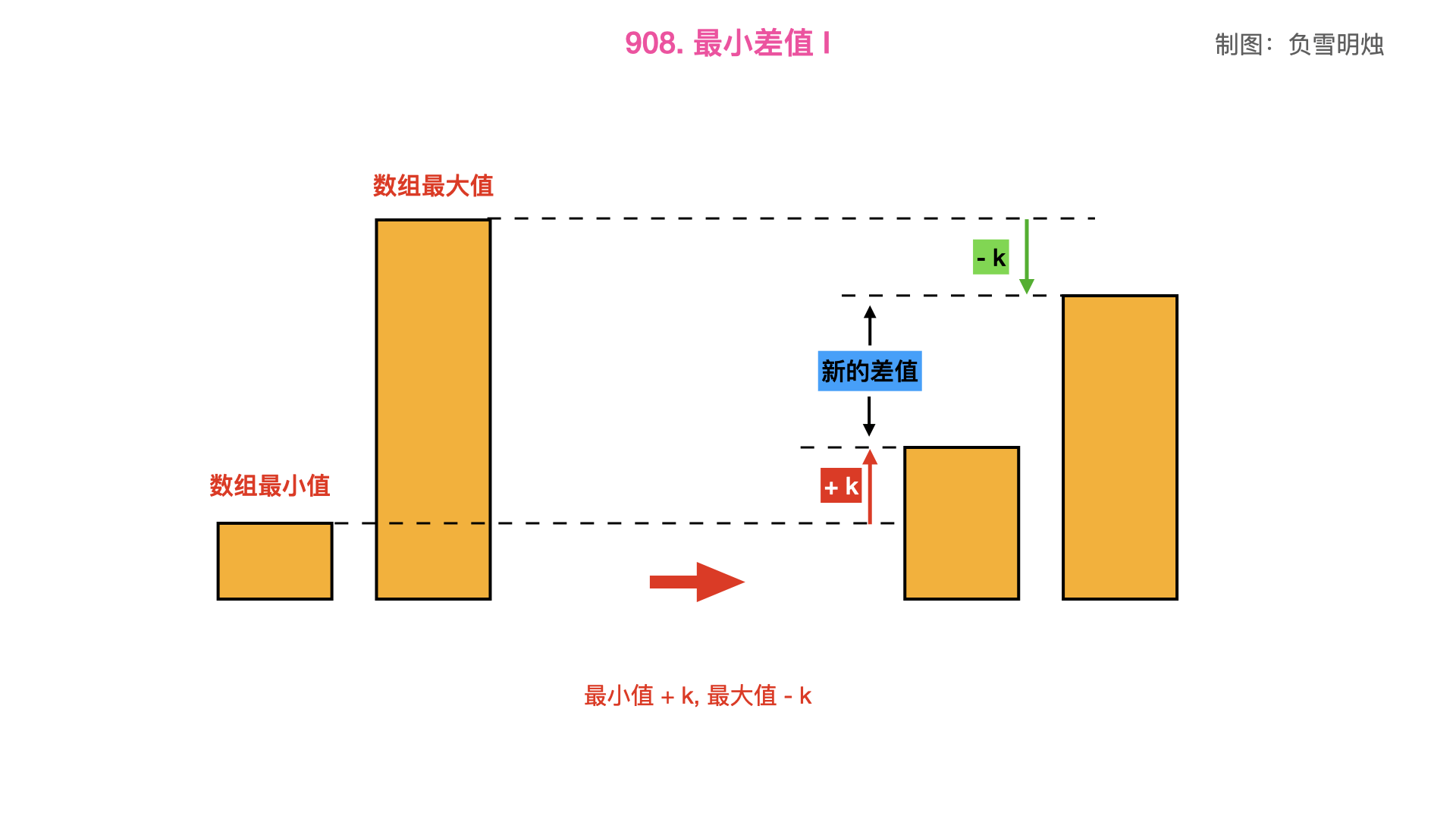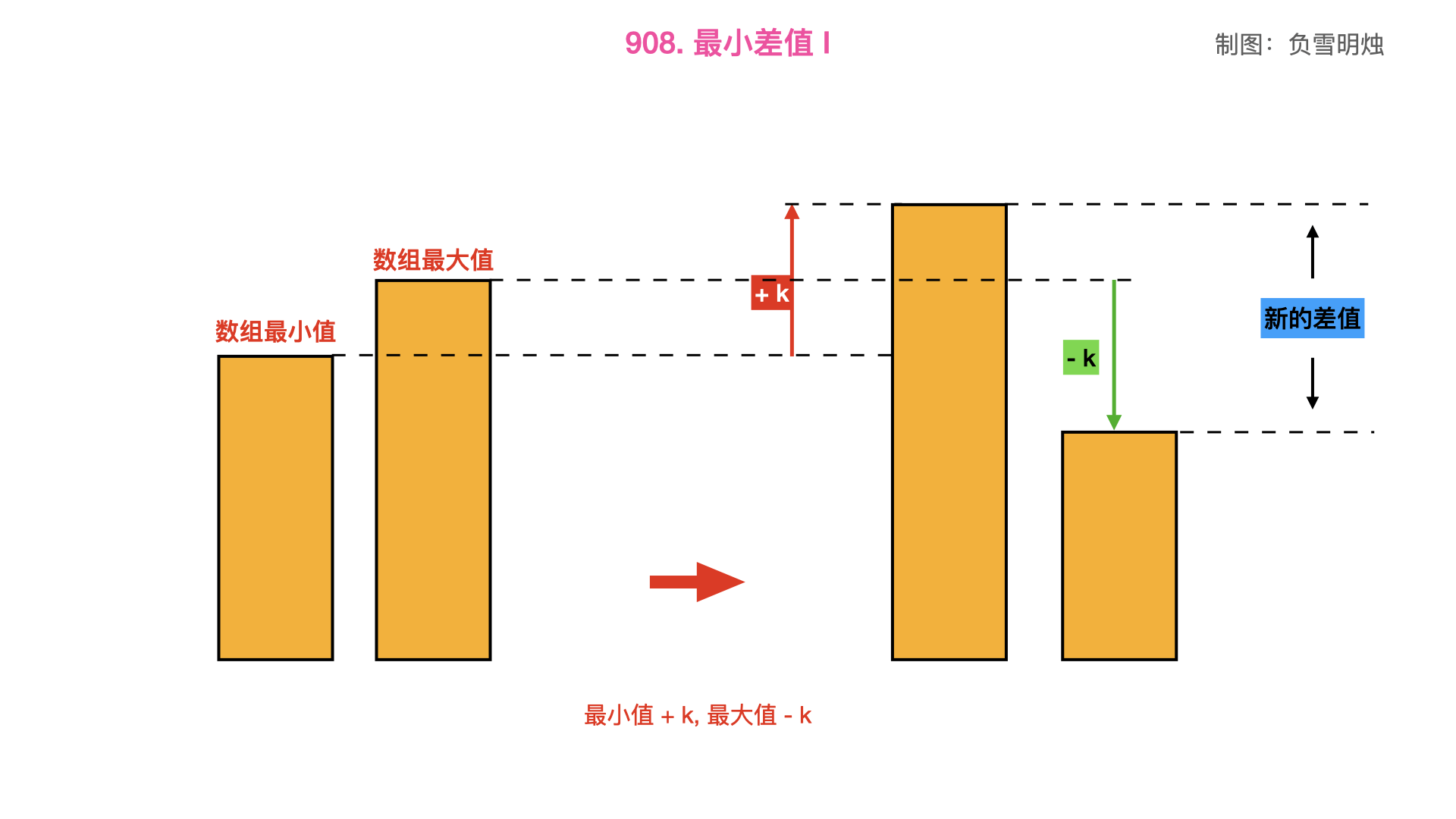• 当原数组的最大值 - 最小值 > 2 * k，那么把最小值 + k，最大值 - k，得到的新数组的最大值和最小值的差最小。
• 否则，得到的新数组的最大值和最小值的差就是 。（不要「矫枉过正」）

class Solution(object):
def smallestRangeI(self, nums, k):
"""
:type nums: List[int]
:type k: int
:rtype: int
"""
diff = max(nums) - min(nums)
if diff > 2 * k:
return diff - 2 * k
return 0


class Solution(object):
def smallestRangeI(self, A, K):
"""
:type A: List[int]
:type K: int
:rtype: int
"""
return max(max(A) - min(A) - 2 * K, 0)


• 时间复杂度：
• 空间复杂度：

## # 总结

1. 对于这种题目，并不需要真正的把“新数组”的每个值都计算出来，只需要求出一个值来，那么一般就是靠思路和规律取胜，不要暴力求“新数组”哦~

## # 日期

2018 年 9 月 23 日 —— 今天是实验室第一个打卡的 2018 年 11 月 5 日 —— 打了羽毛球，有点累